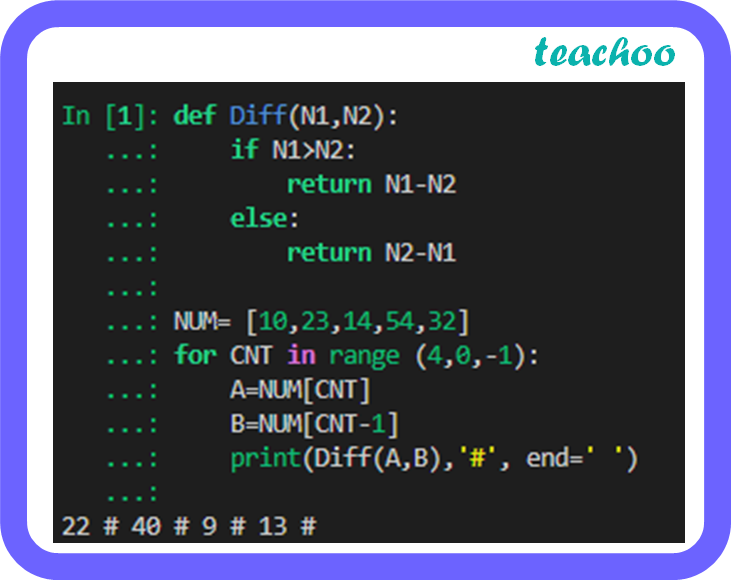CBSE Class 12 Sample Paper for 2023 Boards

Computer Science - Class 12
Solutions to CBSE Sample Paper - Computer Science Class 12

## ``` print(Diff(A,B),'#', end=' ') ```Output: 22 # 40 # 9 # 13 #

Explanation:

• The function Diff( ) takes 2 numbers as parameters and finds the difference by subtracting the smaller number from the larger number and returns the difference.
• NUM is an array of 5 numbers.
• The for loop iterates from 4 to 0 by decrementing by 1.
• A and B are assigned 2 consecutive numbers from the array NUM and the difference is calculated and printed. This is repeated for all 2 consecutive numbers in the array.

Learn in your speed, with individual attention - Teachoo Maths 1-on-1 Class